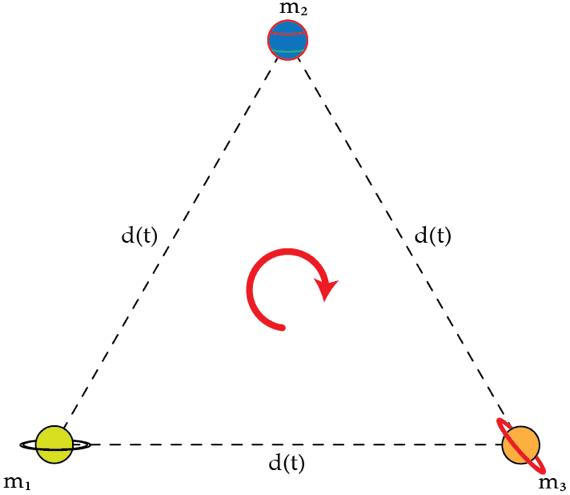# The dance of the planets

Consider three planets of masses $m_{1}= m_{*}, m_{2}=m_{*}/2$ and $m_{3}=m_{*}/3$ located at the vertices of an equilateral triangle with sides of length $d$. It turns out that the planets, under the action of the mutual forces of gravitation, can move in such a way that they always form an equilateral triangle of variable side $d(t)$. Assume that maximum and minimum distances between the planets are $d_{max}=2\times 10^{6}~\text{km}$ and $d_{min}=5\times 10^{5}~\text{km}$ and that after $T=3\times 10^{5}~\text{s}$ the planets return to their original configuration shown in the figure. That is, $T$ is the period of the orbital motion of the planets. Using the information provided, determine the maximum speed in kilometers per second of planet $m_{1}$.Details and assumptions

The universal gravitational constant is $G=6.67\times 10^{-11}~\text{m}^{3}\text{kg}^{-1} \text{s}^{-2}$

×